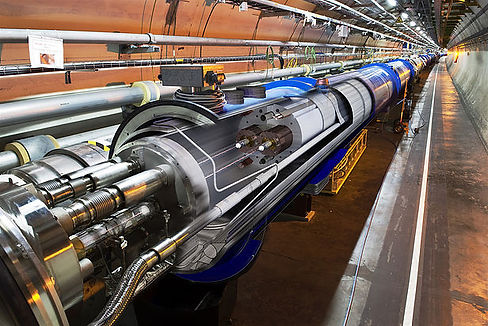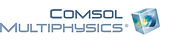top of page

# Computational Physics

Computational Physics is the study and implementation of numerical analysis to solve problems in physics for which a quantitative theory already exists.

In physics, different theories based on mathematical models provide very precise predictions on how systems behave. Unfortunately, it is often the case that solving the mathematical model for a particular system in order to produce a useful prediction is not feasible. In such cases, numerical approximations are required. Computational physics is the subject that deals with these numerical approximations.The huge increase in the power of computers in recent years has made an impact on the role of computational physics. In some cases, entire problems can now be solved computationally without the need for any experimental input. Computer graphics and visualization now play an important role in the scientific process as they can provide greater understanding of physical processes. Advances in microelectronics, numerical analysis and computer science all impact on computational physics and so, it is important that practitioners of the subject are aware of developments in these fields.

Due to the broad class of problems computational physics deals with, it is an essential component of modern research in different areas of physics.## COSY Infinitybottom of page